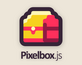# Pixelbox

Create 2D games in JavaScript, made easier · By Cedric Stoquer

# Collision detection with tiles

A topic by prmurphy created Jun 11, 2020 Views: 515 Replies: 4
Viewing posts 1 to 4

As per the title, I'm trying to figure out the best way to detect collision with tiles. I came up with two ideas.

The first is have the main loop always be calling map.get for wherever the character is. and then something like this:

if(map.get(characterXRight, characterYbottom) != null){
if ((map.get(characterX, characterYbottom).sprite == '3') || (map.get(characterXRight, characterYbottom).sprite == '3')) {
//console.log("hello")
if (btn.down){
character.y -= 1
}
}
}

(something like that for all 4 directions.)

The second idea is to use map.find and make an array of all the tiles. Like:

cars = [...map.find(3)];

Which find all tiles of id 3 and add them to the array cars. After that, loop through the array

var i;
for (i = 0; i < cars.length; i++) {

if (AABBcollision(mario, cars[i])==true){
print("Hello, Mario", 12, 10);

}

function AABBcollision(rect1, rect2) {
print(rect2.x * 8, 50,70)
print(rect2.y * 8, 50,80)

print(rect1.x, 40,70)
print(rect1.y, 40,80)

if (rect1.x < (rect2.x * 8) + (rect2.w) &&
rect1.x + rect1.w > (rect2.x * 8) &&
rect1.y < (rect2.y * 8) + (rect2.h) &&
rect1.y + rect1.h > (rect2.y * 8)) {

return true
}

The problem wth this though is that the tilemap returns x.y coordinates based on the tilemap I think I need to multiple by the tile width/height to do the correctly.

I still havent gotten either to work though, so any help would be greatly appreciated.

Ok, I got it working with the array. I hardcoded the pixel width/height and it worked. Lesson learned, dont take anything for granted. So now I have this:

var tileW = settings.tileSize.width || settings.tileSize;
var tileH = settings.tileSize.height || settings.tileSize;
var TileMap  = require('pixelbox/TileMap');
console.log(tileW)
var mario = {
x: 60,
y: 60,
w: tileW,
h: tileH
};
x: 100,
y: 100,
w: tileW,
h: tileH

}
var flipH = "";
var flipV = "";

function AABBcollision(rect1, rect2) {

if (rect1.x < (rect2.x * 8) + (8) &&
rect1.x + rect1.w > (rect2.x * 8) &&
rect1.y < (rect2.y * 8) + (8) &&
rect1.y + rect1.h > (rect2.y * 8)) {

return true
}
}
var walls = []
var map = getMap("mymap");
draw(map,0,0)
//walls = [map.find(4)]
print(walls.length);
exports.update = function () {
if (btn.right) {
mario.x += 1;
flipH = "";
}
if (btn.left) {
mario.x -= 1;
flipH = "flipH";
}
if (btn.up) {
mario.y -= 1;
flipV = "";
}
if (btn.down) {
mario.y += 1;
flipV = "flipV";
}

cls();
draw(map,0,0)

walls = [...map.find(3)];
print(walls.length);
//print(walls.x, 60, 50 )
//print(walls.y, 70, 50 )

sprite(153, mario.x, mario.y, flipH, flipV);

var i;
for (i = 0; i < walls.length; i++) {

if (AABBcollision(mario, walls[i])==true){
print("Hello, Mario", 12, 10);
if (btn.down) {
mario.y -= 0;
mario.y = mario.y - 1
}
if (btn.up) {
mario.y += 1;
}
if (btn.left) {
mario.x += 1;
}
if (btn.right) {
mario.x -= 1;
}

}
}
}

The problem now is I would like the character to be able to move smoothly along the wall when hold down down/left, down/right etc...

At this point it looks like the character is going slightly into the wall a little making it impossible to move. What would be the best way to keep that from happening?

GOT IT! whith the help of https://jonathanwhiting.com/tutorial/collision/

Here's my final code:

var tileW = settings.tileSize.width || settings.tileSize;
var tileH = settings.tileSize.height || settings.tileSize;
var TileMap  = require('pixelbox/TileMap');
var oldY
var oldX
var mario = {
x: 60,
y: 60,
w: tileW,
h: tileH
};
x: 100,
y: 100,
w: tileW,
h: tileH
};
var flipH = "";
var flipV = "";
function AABBcollision(rect1, rect2) {

if (rect1.x < (rect2.x * 8) + (8) &&
rect1.x + rect1.w > (rect2.x * 8) &&
rect1.y < (rect2.y * 8) + (8) &&
rect1.y + rect1.h > (rect2.y * 8)) {
return true
}
}
var walls = []
var map = getMap("mymap");
draw(map,0,0)
print(walls.length);

exports.update = function () {
cls();
draw(map,0,0)
oldY = mario.y
oldX = mario.x
if (btn.right) {
mario.x += 1;
flipH = "";
}
if (btn.left) {
mario.x -= 1;
flipH = "flipH";
}
walls = [...map.find(3)];
var i;
for (i = 0; i < walls.length; i++) {

if (AABBcollision(mario, walls[i])==true){

print("Hello, Mario", 12, 10);
mario.y = oldY
mario.x = oldX
}
}
oldX = mario.x
if (btn.up) {
mario.y -= 1;
//flipV = "";
}
if (btn.down) {
mario.y += 1;
//flipV = "flipV";
}
for (i = 0; i < walls.length; i++) {
if (AABBcollision(mario, walls[i])==true){

print("Hello, Mario", 12, 10);
mario.y = oldY
mario.x = oldX
}
}
sprite(153, mario.x, mario.y, flipH, flipV);
}

Definitely not the most efficient code. I'll have to work on that later. But it is buttery smooth!

thank you so much!!! i've been trying to get basic collision working for a couple days now and you've helped so much

That's awesome! Glad I could help someone.